Next: Specifying initial and boundary Up: Creating or Reading in Previous: Using the triangle libraries   Contents

## Reading triangular grids from a file

Use of the `-t` flag creates three files specified in `suntans.dat`: `points`, `cells`, and `edges`. By default, these are specified to be

```points    points.dat
edges     edges.dat
cells     cells.dat
```
The `points` file contains a listing of the x-y coordinates of the Delaunay points in the full triangulation before being subdivided among different processors. This file contains three columns although the last column is never used. The total number of lines in this file is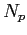, the number of triangle vertics in the triangulation. The `edges` file contains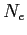rows each of which defines an edge in the triangulation, and five columns in the following format:
```Point1 Point2 Marker Voronoi1 Voronoi2
```
`Point1` and `Point2` contain indices to points in the `points` file and make up the end points of the Delaunay edges. Because SUNTANS uses C-style indexing, then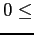`Point1,Point2`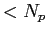. `Marker` specifies the type of edge. If `Marker=0`, then it is a computational edge, otherwise, it is a boundary edge, and the boundary condition is specified in Section 4.2. The last two entries, `Voronoi1` and `Voronoi2`, are the indices to the Voronoi points which make up the end points of the Voronoi edge which intersects this Delaunay edge. As such, we must have`Voronoi1,Voronoi2`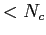. These Voronoi points correspond to triangles defined in the file `cells`. Voronoi points which are ghost points are indicated by a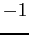. The `cells` file contains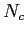rows each of which corresponds to a triangle in the triangulation, and 8 columns in the following format:
```xv yv Point1 Point2 Point3 Neigh1 Neigh2 Neigh3
```
The `xv` and `yv` points correspond to the x-y coordinates of the Voronoi points of each triangle and `Point1`, `Point2`, and `Point3` correspond to indices to points in the `points` file which make up the vertices of the triangle. These indices must satisfy`Point1,Point2,Point3`.
`Neigh1`, `Neigh2`, and `Neigh3` correspond to indices to neighboring triangles. Neighboring triangles which correspond to ghost points are represented by a. For neighbors not lying outside boundaries, we must have`Neigh1,Neigh2,Neigh3`.
Because SUNTANS determines the number of triangle vertices, edges, and cellsby the number of rows in the `points`, `cells`, and `edges` files, respectively, it is important not to have extra carriage returns at the end of these files.

These three files are generated each time the `-t` flag is used with SUNTANS. If the `-t` flag is not used, then when called with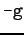, SUNTANS reads these three files and computes grid geometry and, if desired, partitions it among several processors. The `-g` flag outputs the following data files, which are specified in `suntans.dat`. One file associated with each of these descriptors is created for each processor in a partitioned grid. For example, if the file name specified after `cells` in `suntans.dat` is given by `cells.dat`, then when called with 2 processors, the `-g` flag would output two files names `cells.dat.0` and `cells.dat.1`, each corresponding to the `cells` file of each processor.

• `cells` Same as the output when using `-t`, except on a per-processor basis. The indices to the triangle vertices still correspond to indices in the global `points` file, which is not distributed among the processors. All other indices are local to the specific processor.
• `edges` Same as the output when using `-t`, except on a per-processor basis. The indices to the end points of the edges still correspond to indices in the global `points` file, which is not distributed among the processors. All other indices are local to the specific processor.
• `celldata` Contains the grid data associated with the Voronoi points of each cell and containsrows, whereis the number of cells on each processor (including interprocessor ghost points). Each row contains the following entries:
```xv yv Ac dv Nk Edge{1-3} Neigh{1-3} N{1-3} def{1-3}
```
• `xv yv` are the Voronoi coordinates
• `Ac` is the cell area
• `dv` is the depth at the point `xv,yv`. This is the depth of the bottom-most face of the column beneath this cell and is not the actual depth, which is always greater than `dv`.
• `Nk` is the number of vertical levels in the water column.
• `Edge{1-3}` are indices to the three edges that correspond to the faces of the cell.
• `Neigh{1-3}` are the indices to the three neighboring cells.
• `N{1-3}` is the dot product of the unique normal with the outward normal on each face.
• `def{1-3}` is the distance from the Voronoi point to the three faces.
• `edgedata` Contains the grid data associated with the Delaunay edges and containsrows, whereis the number of edges on each processor (including interprocessor edges). Each row contains the following entries:
```df dg n1 n2 xe ye Nke Nkc grad{1,2} gradf{1,2} mark xi{1,2,3,4} eneigh{1,2,3,4}
```
• `df` is the length of the edge.
• `dg` is the distance between the Voronoi points on either side of the edge. If this is a boundary edge then `dg` is twice the distance between the edge and the Voronoi point on the inside of the boundary.
• `n1,n2` are the components of the normal direction of the edge. These correspond to the unique normals of each edge. The outward normal for this edge corresponding to a particular cell is given by `n1*N, n2*N`, where `N` is the dot product of the unique normal with the outward normal and is specified in the `celldata` file.
• `xe, ye` are the coordinates of the intersection of the edge with the Delaunay edge.
• `Nke` is the number of active edges in the vertical (see `Nkc`).
• `Nkc` is the maximum number of active cells in the vertical which neighbor a given edge. `Nkc` is always at least `Nke`. See Figure 1 for a graphical depiction.
 .5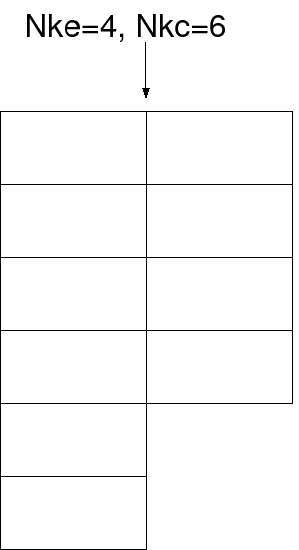item `grad{1,2}` are indices to the Voronoi points defined in the `celldata` file. Ifis the number of cells on a processor, then`grad{1,2}`.
• `gradf{1,2}` are indices that determine the location of the edge in the ordering of the `Edge{1-3}` or `def{1-3}` arrays. Each cell contains a pointer to this edge in its list of `Edge{1-3}` pointers. The `gradf{1,2}` index is a number from 0 to 2 which determines which face number this edge is of a particular cell.
• `mark` Contains the marker type for this edge. All edges with the value 0 are computational edges, while other values are described in Section 4.2.
• `topology`
```Np Nneighs neighbor{0,1,2,...,Np-1}\n
neigh0: num_cells_send num_cells_recv num_edges_send num_edges_recv
cell_send_indices ...
edge_send_indices ...
edge_recv_indices ...
neigh1: num_cells_send num_cells_recv num_edges_send num_edges_recv
cell_send_indices ...
edge_send_indices ...
edge_recv_indices ...
.
.
.
neigh{Numneighs-1}: num_cells_send num_cells_recv num_edges_send num_edges_recv
cell_send_indices ...
• `vertspace` Contains the vertical grid spacings. This file has `Nkmax` rows, where `Nkmax` is the number of z-levels.